# Conversion Formulas

to

## Calculating Total Alkalinity

### What is Alkalinity?

Alkalinity is defined as a measure of the buffering capacity of water to neutralize strong acid. This capacity is attributed to bases that are present in natural waters including OH-, HCO3-, and CO32-. More alkalinity in your water sample means more buffering capacity of your water sample.

In order to determine Alkalinity of your samples, samples will be titrated with sulfuric acid or hydrochloric acid to a certain pH end point (pH 8.3 for Phenolphthalein Alkalinity and pH 4.5 for Total Alkalinity), and the volume of the acid used for the titration will be recorded.

### What is Total Alkalinity?

Total Alkalinity is the amount of acid used to reach pH 4.5. (mL) x 50,000 (mg CaCO3/eq) / sample volume (mL)

Here is an example on how to find Total Alkalinity: 18mL of 0.01M of Sulfuric Acid was required to Titrate 50ml of your sample to pH 4.5.

Total Alkalinity= 18(mL) x 0.02(eq/L) mL acid x 50,000 (mg CaCO3/eq) / 50(mL) = 360(mg/L)

Total Alkalinity: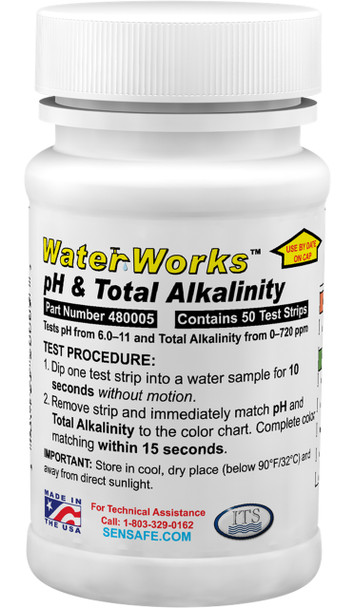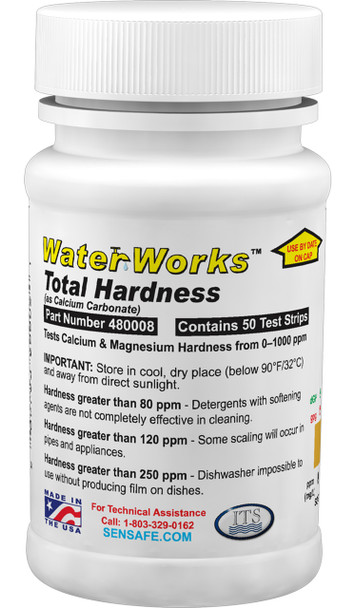## Water Hardness

### Water Hardness Levels

Soft: 0 to 75 mg/L as CaCO3

Moderate: 76 to 150 mg/L as CaCO3

Hard: 151 to 300mg/L as CaCO3

Very Hard: Above 301 mg/L as CaCO3

Total Hardness = Calcium Hardness + Magnesium Hardness

Water should have a total hardness of less than 75 to 85mg/l as CaCO3 and a magnesium hardness of less than 40mg/l as CaCO3 to minimize scaling at elevated temperatures.

Total Hardness:

## Percent Transmittance & Absorbance

### Percent Transmittance(%T) to Absorbance

To convert a value from percent transmittance (%T) to absorbance, use the following equation:
Absorbance = 2 - log(%T)

• Example: convert 56%T to absorbance
• 2 - log(56) = 0.252 absorbance unit
Absorbance

### Absorbance to Percent Transmittance

To convert a value from absorbance to percent transmittance, use the following equation:
%T = antilog (2 - absorbance)

• Example: convert an absorbance of 0.505 to %T
• antilog (2 - 0.505) = 31.3 %T
%T

## Grains

### What is a grain per gallon (gpg)?

One grain is equivalent to 64.799 milligrams. It's a historic unit of weight once used for barleycorn. A grain per gallon is the weight of a substance in one gallon of water, similar to a milligram per liter.

### Converting gpg to mg/L

Formula: gpg x 17.1 = mg/L

Example: 3gpg x 17.1 = 51mg/L

Converts to: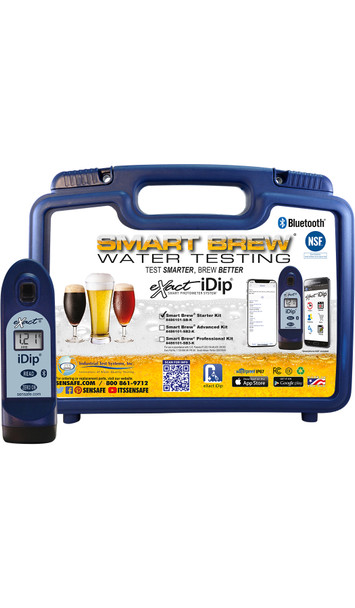### calcium carbonate ppm (CaCO3)

Converts to:
Calcium Ion (Ca+2):
Magnesium Ion (Mg+2):
Magnesium Chloride (MgCl2):
Grain Per Gallon (gpg):
Hardness
Degrees of General Hardness (dGH):
French Hardness (FH):
English Hardness (EH):

### chlorine dioxide ppm (as ClO2) to chlorine (as Cl2)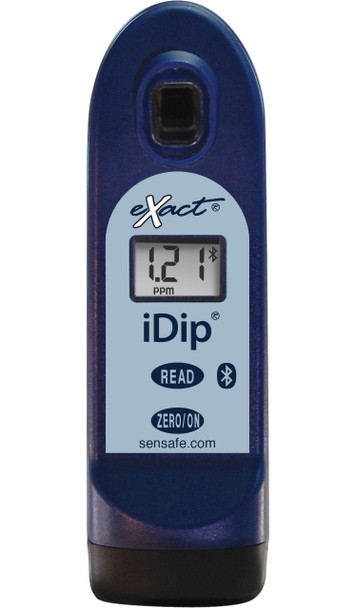### chlorine ppm (as Cl2) to mono chloramines ppm

Formula: ppm (Cl2) x 0.7259

hexavalent chromium ppm (as Cr+6)

to

manganese (Mn+2)

to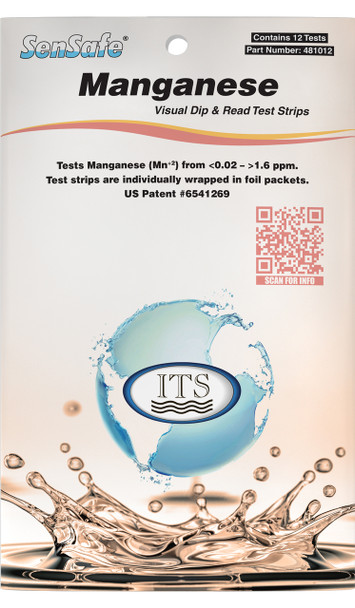-->